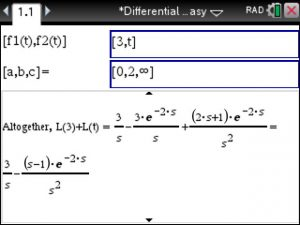# TiNspire: LaPlace Transforms of a Piecewise-Defined Function

Laplace transform over Piecewise def. Function

Example:

f(1) = 3 defined over 0<= t <2

f(2) = t  defined over t >= 2

To find the LaPlace Transform use Differential Equations Made Easy at

https://www.tinspireapps.com/?a=DEQME  and select LAPLACE TRANSFORM OF PIECEWISE DEFINED FUNCTION and enter as follows:We use infinity since the function f2 is not bounded. If it was bounded by for example 10 then we would have entered as [0,2,10]

Posted on Categories differential equation, laplace transform